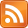# Quaternion Cheatsheet

02 Mar 2012, 17:55 UTC

A quaternion has a vector part and a scalar part. We'll denote the scalar part 'r' and the vector part 'V':

``````quat = (r,V)
``````

Quaternion multiplication is defined as follows:

``````q1 = (r1,V1)
q2 = (r2,V2)
q1*q2 = (r',V') where:
r' = r1.r2 - V1.V2
V' = r1.V2 + r2.V1 + V1^V2
``````

If quat is a unit quaternion then:

``````inverse quat = (r,-V)
``````

To rotate a vector X by a quaternion we work as follows:

``````position quat = (0,X)
resulting position quat = (r,V) * (0,X) * (r,-V)
= (0,X')
``````

Working through using the multiplication formula gives vector X rotated by quaternion (r,V) as follows:

``````X' = (r.r - V.V)X + 2(V.X)V + 2r(V^X)
``````

As a rough guide:

• Matrix3 * Vector3 = 9 operations
• Quat * Vector3 = 28 operations
• Quat -> Matrix3 = 30 operations

To multiply different numbers of vectors:

1. quats 28 matrices 39
2. quats 56 matrices 48
3. quats 84 matrices 57

ivan commented:

I'm not quite sure what <dots> in second code area means? I'd also like to know where quaternions can be used.

on 06 Jun 2012, 10:26 UTC

Eddie Edwards commented:

Ivan, the dots mean multiplication or dot product. So r1.r2 and rN.vM just multiplies; V1.V2 is a dot product.

Quaternions are useful to represent 3D rotations without the full weight of the traditional 3x3 matrix. Advantages are smaller storage space (very important inside a GPU program) and fewer spare degrees of freedom.

As a unit quaternion has 3DOF, and 3D rotations have 3DOF, they map very nicely. Renormalization of a non-unit quaternion is an easy way to remove the extra DOF inherent in the storage format (4DOF), for instance if you operate on some quaternions and get not-quite-precise results. If you have a 3x3 matrix (9DOF) and you know it's a rotation plus errors, it's highly non-trivial to normalize it into a correct orthonormal form.

For example, using a 3x3 matrix as an accumulator and multiplying every frame by a small rotation will eventually give you a matrix which somehow warps your object, and it's hard to remove the warping from the matrix. Using a quaternion as an accumulator in the same way, all you have to do is normalize it back to a unit quaternion as errors accumulate. The result is still slightly "wrong", but it is a valid rotation.

on 07 Jun 2012, 08:06 UTC updated 07 Jun 2012, 08:07 UTC